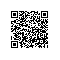# Range Types

range types可以用来标识一些类型（range's subtype）的范围。例如：ranges of timestamp可以用来表示一个会议室预定的起始和结束时间，对应的类型为tsrange，而timestamp为tsrange的subtype。subtype必须要能够以某种方式来排序，这样才能对某个值确定是在range范围内，还是在之前或之后。
range type十分有效，因为它仅用一个值就可以表示在某个范围内的一连串值，而且对于overlapping ranges的概念，range type也可以简洁清楚的表达出来。使用range type的常见场景如：time and date range在会议议程上的安排。

# 当前内置支持的range类型

• int4range — Range of integer
• int8range — Range of bigint
• numrange — Range of numeric
• tsrange — Range of timestamp without time zone
• tstzrange — Range of timestamp with time zone
• daterange — Range of date

# 使用案例

CREATE TABLE reservation (room int, during tsrange);

INSERT INTO reservation VALUES
(1108, '[2010-01-01 14:30, 2010-01-01 15:30)');

select * from reservation;
room |                    during
------+-----------------------------------------------
1108 | ["2010-01-01 14:30:00","2010-01-01 15:30:00")

-- Containment
postgres=> SELECT int4range(10, 20) @> 3;
?column?
----------
f
postgres=> SELECT int4range(10, 20) @> 11;
?column?
----------
t
(1 行记录)

-- Overlaps
postgres=> SELECT numrange(11.1, 22.2) && numrange(20.0, 30.0);
?column?
----------
t

-- Extract the upper bound
postgres=> SELECT upper(int8range(15, 25));
upper
-------
25

postgres=> SELECT int4range(10, 20) * int4range(15, 25);
?column?
----------
[15,20)

-- Is the range empty?
postgres=> SELECT isempty(numrange(1, 5));
isempty
---------
f
(1 行记录)

# 上下限

range类型的输入值必须符合以下几种模式：

(lower-bound,upper-bound)
(lower-bound,upper-bound]
[lower-bound,upper-bound)
[lower-bound,upper-bound]
empty

-- includes 3, does not include 7, and does include all points in between
SELECT '[3,7)'::int4range;

-- does not include either 3 or 7, but includes all points in between
SELECT '(3,7)'::int4range;

-- includes only the single point 4
SELECT '[4,4]'::int4range;

-- includes no points (and will be normalized to 'empty')
SELECT '[4,4)'::int4range;

# 构建range类型

-- The full form is: lower bound, upper bound, and text argument indicating
-- inclusivity/exclusivity of bounds.
SELECT numrange(1.0, 14.0, '(]');
-- If the third argument is omitted, '[)' is assumed.
SELECT numrange(1.0, 14.0);
-- Although '(]' is specified here, on display the value will be converted to
-- canonical form, since int8range is a discrete range type (see below).
SELECT int8range(1, 14, '(]');
-- Using NULL for either bound causes the range to be unbounded on that side.
SELECT numrange(NULL, 2.2);

# range类型操作运算符和函数

range类型支持的运算符如下：

= equal int4range(1,5) = '[1,4]'::int4range t
<> not equal numrange(1.1,2.2) <> numrange(1.1,2.3) t
< less than int4range(1,10) < int4range(2,3) t
< greater than int4range(1,10) > int4range(1,5) t
@> contains range int4range(2,4) @> int4range(2,3) t
@> contains element '[2011-01-01,2011-03-01)'::tsrange @> '2011-01-10'::timestamp t
<@ range is contained by int4range(2,4) <@ int4range(1,7) t
<@ element is contained by 42 <@ int4range(1,7) f
&& overlap (have points in common) int8range(3,7) && int8range(4,12) t
<< strictly left of int8range(1,10) << int8range(100,110) t
>> strictly right of int8range(50,60) >> int8range(20,30) t
&< does not extend to the right of int8range(1,20) &< int8range(18,20) t
&> does not extend to the left of int8range(7,20) &> int8range(5,10) t
-|- is adjacent to numrange(1.1,2.2) -|- numrange(2.2,3.3) t
+ union numrange(5,15) + numrange(10,20) [5,20)
* intersection int8range(5,15) * int8range(10,20) [10,15)
- difference int8range(5,15) - int8range(10,20) [5,10)

Union和difference运算符在得出的结构如果包含两个不重叠的子range（也就是说最终结果无法用一个有效的range类型表示），那么这个运算最后会直接报错。

lower(anyrange) range's element type lower bound of range lower(numrange(1.1,2.2)) 1.1
upper(anyrange) range's element type upper bound of range upper(numrange(1.1,2.2)) 2.2
isempty(anyrange) boolean is the range empty? isempty(numrange(1.1,2.2)) false
lower_inc(anyrange) boolean is the lower bound inclusive? lower_inc(numrange(1.1,2.2)) true
upper_inc(anyrange) boolean is the upper bound inclusive? upper_inc(numrange(1.1,2.2)) false
lower_inf(anyrange) boolean is the lower bound infinite? lower_inf('(,)'::daterange) true
upper_inf(anyrange) boolean is the upper bound infinite? upper_inf('(,)'::daterange) true

# 离散的range类型

ADB PG 6.0内置的range类型int4range, int8range, and daterange都指定了canonicalization函数。

# 自定义新的range类型

CREATE TYPE floatrange AS RANGE (
subtype = float8,
subtype_diff = float8mi
);

SELECT '[1.234, 5.678]'::floatrange;

# 索引

CREATE INDEX reservation_idx ON reservation USING gist (during);

GiST或SP-GiST索引可以来加速涉及range算子操作的查询，如：=, &&, <@, @>, <<, >>, -|-, &<, and &。

# 参考资料